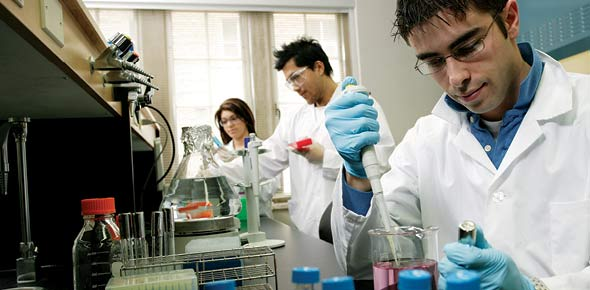# Biochem Introduction

10 QuestionsSettingsIntroduction to biochem. 10 question

Related Topics
• 1.
• A.

Hydrogen bonds

• B.

Covalent bonds

• C.

Ionic bonds

• D.

Van der waals interaction

• 2.
What is the main property of enzymes?
• A.

They are steroselective

• B.

They are hydrophobic

• C.

They are lipophilic

• D.

None of the above

• 3.
• A.

Transferase

• B.

Carboxylase

• C.

Light

• D.

ATP

• 4.
Which of the following is a selective serotonin reuptake inhibitor (SSRI) antidepressant?
• A.

Lexapro

• B.

Celexa

• C.

Retinal

• D.

None of the above

• 5.
• A.

Nitric oxide

• B.

Propane

• C.

Carbon dioxide

• D.

Water

• 6.
The partial negative charge on the oxygen and partial positive charge on hydrogen makes water a polar solvent and enables what?
• A.

Hydrogen bonds

• B.

Convalent bonds

• C.

Ionic bonds

• D.

Van der waals interactions

• 7.
The dielectric constant of water is?
• A.

A high constant

• B.

A low constant

• C.

Not yet been determined

• D.

• 8.
The dielectric constant of a non polar solvent is?
• A.

A high constant

• B.

A low constant

• C.

Has not yet been deterimined

• D.

• 9.
• A.

1 Osmoles

• B.

2 Osmoles

• C.

3 Osmoles

• D.

4 Osmoles

• 10.
• A.

4.6

• B.

7.4

• C.

10.3

• D.

12.7